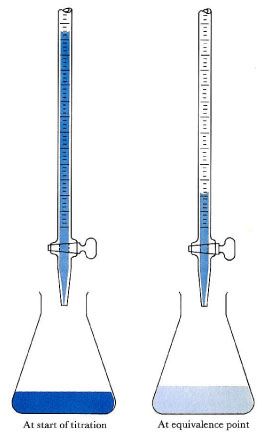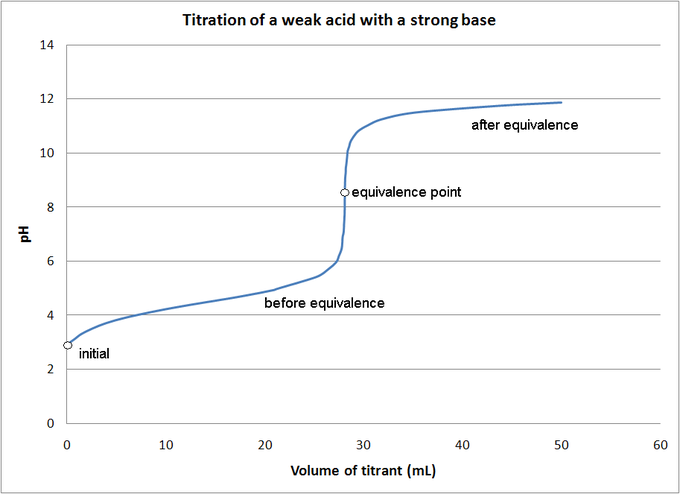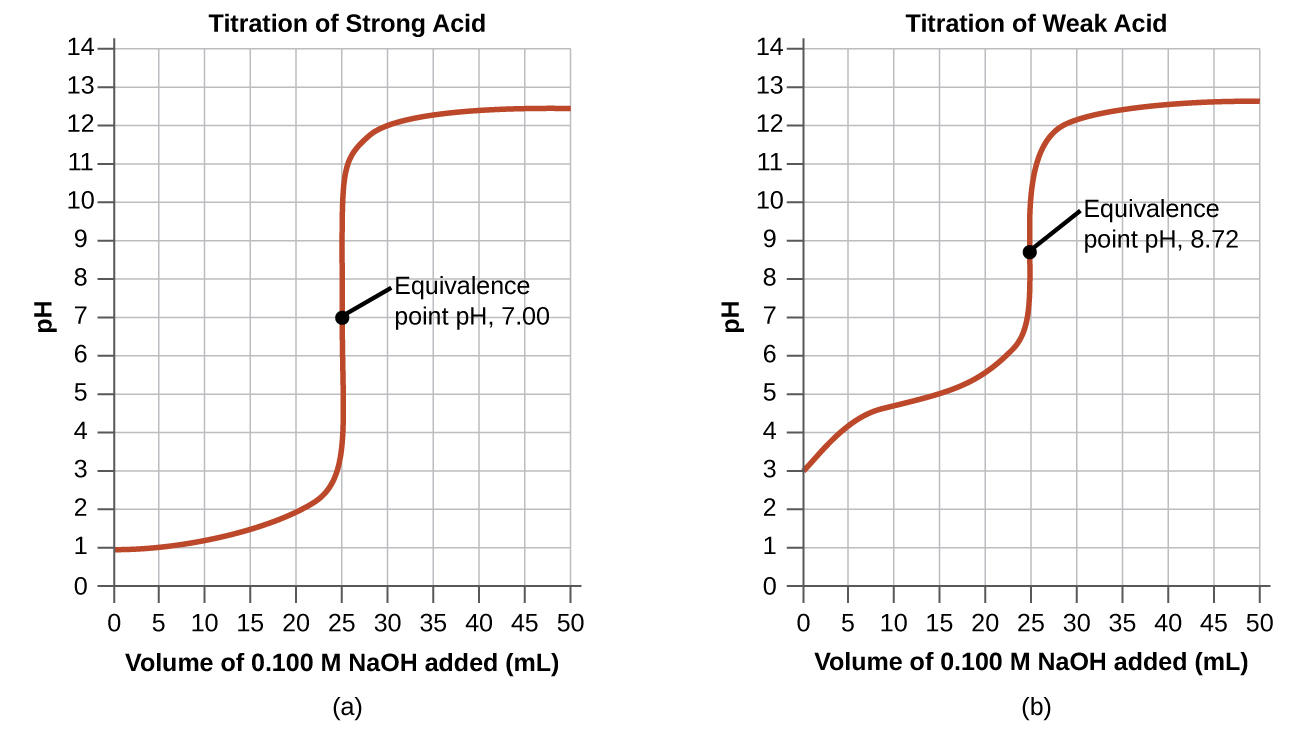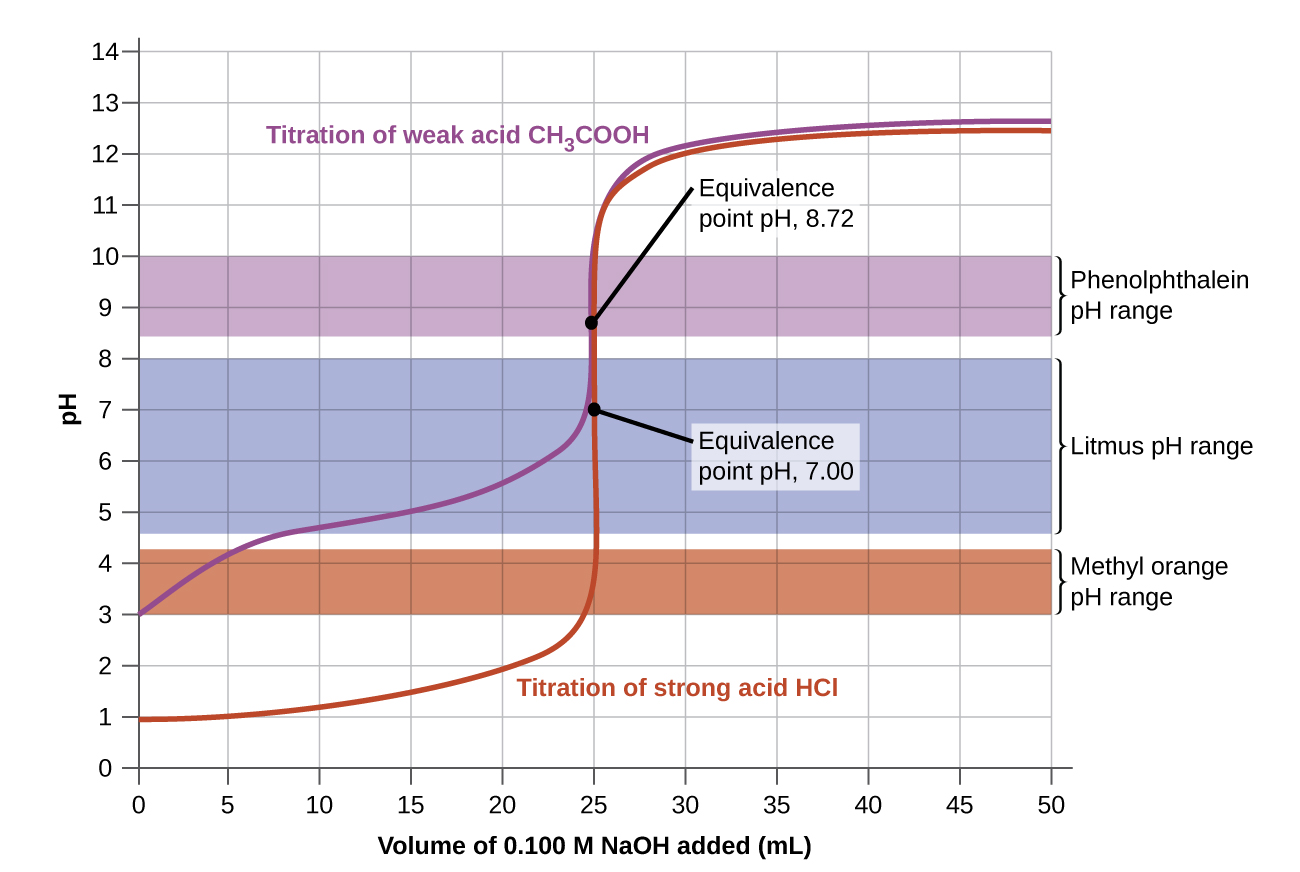# ACID–BASE TITRATIONS

• acid-base titrationdetermines the concentration of an acid or base by exactly neutralizing it with an acid or base of known concentration
• equivalence pointthe point at which an added titrant’s moles are stoichiometrically equal to the moles of acid/base in the sample; the smallest amount of titrant needed to fully neutralize or react with the analyte
• titrantthe standardized (known) solution (either an acid or a base) that is added during titration
• analytethe unknown solution whose concentration is being determined in the titration

## Setting up an Acid-Base Titration

An acid-base titration is an experimental procedure used to determined the unknown concentration of an acid or base by precisely neutralizing it with an acid or base of known concentration. This lets us quantitatively analyze the concentration of the unknown solution. Acid-base titrations can also be used to quantify the purity of chemicals.Acid-base titrationThe solution in the flask contains an unknown number of equivalents of base (or acid). The burette is calibrated to show volume to the nearest 0.001 cm3. It is filled with a solution of strong acid (or base) of known concentration. Small increments are added from the burette until, at the end point, one drop changes the indicator color permanently. (An indication of the approaching equivalence point is the appearance, and disappearance after stirring, of the color that the indicator assumes beyond neutralization.) At the equivalence point, the total amount of acid (or base) is recorded from the burette readings. The number of equivalents of acid and base must be equal at the equivalence point.

Alkalimetry, or alkimetry, is the specialized analytic use of acid-base titration to determine the concentration of a basic (alkaline) substance; acidimetry, or acidometry, is the same concept applied to an acidic substance.

## Materials for a Titration Procedure

• burette
• white tile (used to see a color change in the solution)
• pipette
• pH indicator (the type depends on the reactants)
• titrant (a standard solution of known concentration; a common example is aqueous sodium carbonate)
• analyte, or titrand (the solution of unknown concentration)

## Equivalence Point Indicators

Before you begin the titration, you must choose a suitable pH indicator, preferably one that will experience a color change (known as the “end point”) close to the reaction’s equivalence point; this is the point at which equivalent amounts of the reactants and products have reacted. Below are some common equivalence point indicators:

• strong acid-strong base titration: phenolphthalein indicator
• weak acid-weak base titration: bromthymol blue indicator
• strong acid-weak base titration: methyl orange indicator the base is off the scale (e.g., pH > 13.5) and the acid has pH > 5.5: alizarine yellow indicator
• the base is off the scale (e.g., pH > 13.5) and the acid has pH > 5.5: alizarine yellow indicator
• the base is off the scale (e.g., pH > 13.5) and the acid has pH > 5.5: alizarine yellow indicator
• the acid is off the scale (e.g., pH < 0.5) and the base has pH < 8.5: thymol blue indicator

## Estimating the Equivalence Point’s pH

The resulting solution at the equivalence point will have a pH dependent on the acid and base’s relative strengths. You can estimate the equivalence point’s pH using the following rules:

• A strong acid will react with a weak base to form an acidic (pH < 7) solution.
• A strong acid will react with a strong base to form a neutral (pH = 7) solution.
• A weak acid will react with a strong base to form a basic (pH > 7) solution.

When a weak acid reacts with a weak base, the equivalence point solution will be basic if the base is stronger and acidic if the acid is stronger; if both are of equal strength, then the equivalence pH will be neutral. Weak acids are not often titrated against weak bases, however, because the color change is brief and therefore very difficult to observe.

You can determine the pH of a weak acid solution being titrated with a strong base solution at various points; these fall into four different categories: (1) initial pH; (2) pH before the equivalence point; (3) pH at the equivalence point; and (4) pH after the equivalence point.Titration of a weak acid by a strong baseThe pH of a weak acid solution being titrated with a strong base solution can be found at each indicated point.

## Titration Procedure

1. Rinse the burette with the standard solution, the pipette with the unknown solution, and the conical flask with distilled water.
2. Place an accurately measured volume of the analyte into the Erlenmeyer flask using the pipette, along with a few drops of indicator. Place the standardized solution into the burette, and indicate its initial volume in a lab notebook. At this stage, we want a rough estimate of the amount of known solution necessary to neutralize the unknown solution. Let the solution out of the burette until the indicator changes color, and record the value on the burette. This is the first titration and it is not very precise; it should be excluded from any calculations.
3. Perform at least three more titrations, this time more accurately, taking into account where the end point will roughly occur. Record the initial and final readings on the burette, prior to starting the titration and at the end point, respectively. (Subtracting the initial volume from the final volume will yield the amount of titrant used to reach the endpoint.)
4. The end point is reached when the indicator permanently changes color.

In the example, we calculated pH at four points during a titration. shows a detailed sequence of changes in the pH of a strong acid and a weak acid in a titration with NaOH.

Volume of 0.100 M NaOH Added (mL) Moles of NaOH Added pH Values 0.100 M HCl pH Values 0.100 MCH3CO2H
0.0 0.0 1.00 2.87
5.0 0.00050 1.18 4.14
10.0 0.00100 1.37 4.57
15.0 0.00150 1.60 4.92
20.0 0.00200 1.95 5.35
22.0 0.00220 2.20 5.61
24.0 0.00240 2.69 6.13
24.5 0.00245 3.00 6.44
24.9 0.00249 3.70 7.14
25.0 0.00250 7.00 8.72
25.1 0.00251 10.30 10.30
25.5 0.00255 11.00 11.00
26.0 0.00260 11.29 11.29
28.0 0.00280 11.75 11.75
30.0 0.00300 11.96 11.96
35.0 0.00350 12.22 12.22
40.0 0.00400 12.36 12.36
45.0 0.00450 12.46 12.46
50.0 0.00500 12.52 12.52
Table 4. pH Values in the Titrations of a Strong Acid with a Strong Base and of a Weak Acid with a Strong Base

The simplest acid-base reactions are those of a strong acid with a strong base.  shows data for the titration of a 25.0-mL sample of 0.100 M hydrochloric acid with 0.100 M sodium hydroxide. The values of the pH measured after successive additions of small amounts of NaOH are listed in the first column of this table, and are graphed in a form that is called a titration curve. The pH increases slowly at first, increases rapidly in the middle portion of the curve, and then increases slowly again. The point of inflection (located at the midpoint of the vertical part of the curve) is the equivalence point for the titration. It indicates when equivalent quantities of acid and base are present. For the titration of a strong acid with a strong base, the equivalence point occurs at a pH of 7.00 and the points on the titration curve can be calculated using solution stoichiometryFigure 1. (a) The titration curve for the titration of 25.00 mL of 0.100 M HCl (strong acid) with 0.100 M NaOH (strong base) has an equivalence point of 7.00 pH. (b) The titration curve for the titration of 25.00 mL of 0.100 M HCl (strong acid) with 0.100 M NaOH (strong base) has an equivalence point of 8.72 pH.

The titration of a weak acid with a strong base (or of a weak base with a strong acid) is somewhat more complicated than that just discussed, but it follows the same general principles. Let us consider the titration of 25.0 mL of 0.100 M acetic acid (a weak acid) with 0.100 M sodium hydroxide and compare the titration curve with that of the strong acid. Table 4 gives the pH values during the titration, Figure 1 shows the titration curve.

Although the initial volume and molarity of the acids are the same, there are important differences between the two titration curves. The titration curve for the weak acid begins at a higher value (less acidic) and maintains higher pH values up to the equivalence point. This is because acetic acid is a weak acid, which is only partially ionized. The pH at the equivalence point is also higher (8.72 rather than 7.00) due to the hydrolysis of acetate, a weak base that raises the pH:$\text{CH}_3\text{CO}_2^{\;\;-}(aq)\;+\;\text{H}_2\text{O}(l)\;{\leftrightharpoons}\;\text{CH}_3\text{CO}_2\text{H}(l)\;+\;\text{OH}^{-}(aq)$

After the equivalence point, the two curves are identical because the pH is dependent on the excess of hydroxide ion in both cases.

# Acid-Base Indicators

Certain organic substances change color in dilute solution when the hydronium ion concentration reaches a particular value. For example, phenolphthalein is a colorless substance in any aqueous solution with a hydronium ion concentration greater than 5.0 × 10−9M (pH < 8.3). In more basic solutions where the hydronium ion concentration is less than 5.0 × 10−9M(pH > 8.3), it is red or pink. Substances such as phenolphthalein, which can be used to determine the pH of a solution, are called acid-base indicators. Acid-base indicators are either weak organic acids or weak organic bases.

The equilibrium in a solution of the acid-base indicator methyl orange, a weak acid, can be represented by an equation in which we use HIn as a simple representation for the complex methyl orange molecule:$\begin{array}{ccccccc} \text{HIn}(aq) & + & \text{H}_2\text{O}(l) & {\leftrightharpoons} & \text{H}_3\text{O}^{+}(aq) & + & \text{In}^{-}(aq) \\[0.5em] \text{red} & & & & & & \text{yellow} \end{array}$$K_{\text{a}} = \frac{[\text{H}_3\text{O}^{+}][\text{In}^{-}]}{[\text{HIn}]} = 4.0\;\times\;10^{-4}$

The anion of methyl orange, In, is yellow, and the nonionized form, HIn, is red. When we add acid to a solution of methyl orange, the increased hydronium ion concentration shifts the equilibrium toward the nonionized red form, in accordance with Le Châtelier’s principle. If we add base, we shift the equilibrium towards the yellow form. This behavior is completely analogous to the action of buffers.

An indicator’s color is the visible result of the ratio of the concentrations of the two species Inand HIn. If most of the indicator (typically about 60−90% or more) is present as In, then we see the color of the In ion, which would be yellow for methyl orange. If most is present as HIn, then we see the color of the HIn molecule: red for methyl orange. For methyl orange, we can rearrange the equation for Ka and write:$\frac{[\text{In}^{-}]}{[\text{HIn}]} = \frac{[\text{substance\;with\;yellow\;color}]}{[\text{substance\;with\;red\;color}]} = \frac{K_{\text{a}}}{[\text{H}_3\text{O}^{+}]}$

This shows us how the ratio of$\frac{[\text{In}^{-}]}{[\text{HIn}]}$ varies with the concentration of hydronium ion.

The above expression describing the indicator equilibrium can be rearranged:$\frac{[\text{H}_3\text{O}^{+}]}{K_{\text{a}}} = \frac{[\text{HIn}]}{[\text{In}^{-}]}$$\text{log}(\frac{[\text{H}_3\text{O}^{+}]}{K_{\text{a}}}) = \text{log}(\frac{[\text{HIn}]}{[\text{In}^{-}]})$$\text{log}([\text{H}_3\text{O}^{+}])\;-\;\text{log}(K_{\text{a}}) = -\text{log}(\frac{[\text{In}^{-}]}{[\text{HIn}]})$$-\text{pH}\;+\;\text{p}K_{\text{a}} = -\text{log}(\frac{[\text{In}^{-}]}{[\text{HIn}]})$$\text{pH} = \text{p}K_{\text{a}}\;+\;\text{log}(\frac{[\text{In}^{-}]}{[\text{HIn}]})\;\text{or\;pH} = \text{p}K_{\text{a}}\;+\;\text{log}(\frac{[\text{base}]}{[\text{acid}]})$

The last formula is the same as the Henderson-Hasselbalch equation, which can be used to describe the equilibrium of indicators.

When [H3O+] has the same numerical value as Ka, the ratio of [In] to [HIn] is equal to 1, meaning that 50% of the indicator is present in the red form (HIn) and 50% is in the yellow ionic form (In), and the solution appears orange in color. When the hydronium ion concentration increases to 8 × 10−4M (a pH of 3.1), the solution turns red. No change in color is visible for any further increase in the hydronium ion concentration (decrease in pH). At a hydronium ion concentration of 4 × 10−5M (a pH of 4.4), most of the indicator is in the yellow ionic form, and a further decrease in the hydronium ion concentration (increase in pH) does not produce a visible color change. The pH range between 3.1 (red) and 4.4 (yellow) is the color-change interval of methyl orange; the pronounced color change takes place between these pH values.

There are many different acid-base indicators that cover a wide range of pH values and can be used to determine the approximate pH of an unknown solution by a process of elimination. Universal indicators and pH paper contain a mixture of indicators and exhibit different colors at different pHs. Figure 2 presents several indicators, their colors, and their color-change intervals.

Titration curves help us pick an indicator that will provide a sharp color change at the equivalence point. The best selection would be an indicator that has a color change interval that brackets the pH at the equivalence point of the titration.

The color change intervals of three indicators are shown below. The equivalence points of both the titration of the strong acid and of the weak acid are located in the color-change interval of phenolphthalein. We can use it for titrations of either strong acid with strong base or weak acid with strong base.Figure 3. The graph shows a titration curve for the titration of 25.00 mL of 0.100 M CH3CO2H (weak acid) with 0.100 MNaOH (strong base) and the titration curve for the titration of HCl (strong acid) with NaOH (strong base). The pH ranges for the color change of phenolphthalein, litmus, and methyl orange are indicated by the shaded areas.

Litmus is a suitable indicator for the HCl titration because its color change brackets the equivalence point. However, we should not use litmus for the CH3CO2H titration because the pH is within the color-change interval of litmus when only about 12 mL of NaOH has been added, and it does not leave the range until 25 mL has been added. The color change would be very gradual, taking place during the addition of 13 mL of NaOH, making litmus useless as an indicator of the equivalence point.

We could use methyl orange for the HCl titration, but it would not give very accurate results: (1) It completes its color change slightly before the equivalence point is reached (but very close to it, so this is not too serious); (2) it changes color, as Figure 3 shows, during the addition of nearly 0.5 mL of NaOH, which is not so sharp a color change as that of litmus or phenolphthalein; and (3) it goes from yellow to orange to red, making detection of a precise endpoint much more challenging than the colorless to pink change of phenolphthalein. Figure 3shows us that methyl orange would be completely useless as an indicator for the CH3CO2H titration. Its color change begins after about 1 mL of NaOH has been added and ends when about 8 mL has been added. The color change is completed long before the equivalence point (which occurs when 25.0 mL of NaOH has been added) is reached and hence provides no indication of the equivalence point.

We base our choice of indicator on a calculated pH, the pH at the equivalence point. At the equivalence point, equimolar amounts of acid and base have been mixed, and the calculation becomes that of the pH of a solution of the salt resulting from the titration.

EXAMPLE

### Titration Problem

A 25 ml solution of 0.5 M NaOH is titrated until neutralized into a 50 ml sample of HCl. What was the concentration of the HCl?

Step-by-Step Solution

Step 1 – Determine [OH]
Every mole of NaOH will have one mole of OH. Therefore [OH] = 0.5 M.
Step 2 – Determine the number of moles of OH
Molarity = # of moles/volume
# of moles = Molarity x Volume
# of moles OH = (0.5 M)(.025 L)
# of moles OH = 0.0125 mol
Step 3 – Determine the number of moles of H+
When the base neutralizes the acid, the number of moles of H+ = the number of moles of OH. Therefore the number of moles of H+ = 0.0125 moles.
Step 4 – Determine the concentration of HCl
Every mole of HCl will produce one mole of H+, therefore the number of moles of HCl = number of moles of H+.
Molarity = # of moles/volume
Molarity of HCl = (0.0125 mol)/(0.050 L)
Molarity of HCl = 0.25 M
The concentration of the HCl is 0.25 M.
Another Solution Method
The above steps can be reduced to one equation
MacidVacid = MbaseVbase
where
Macid = concentration of the acid
Vacid = volume of the acid

Mbase = concentration of the base
Vbase = volume of the base

This equation works for acid/base reactions where the mole ratio between acid and base is 1:1. If the ratio were different as in Ca(OH)2 and HCl, the ratio would be 1 mole acid to 2 moles base. The equation would now be
MacidVacid = 2MbaseVbase
For the example problem, the ratio is 1:1
MacidVacid = MbaseVbase
Macid(50 ml)= (0.5 M)(25 ml)

Macid = 12.5 MmL/50 ml
Macid = 0.25 M

## STEM Elearning

We at FAWE have built this platform to aid learners, trainers and mentors get practical help with content, an interactive platform and tools to power their teaching and learning of STEM subjects, more